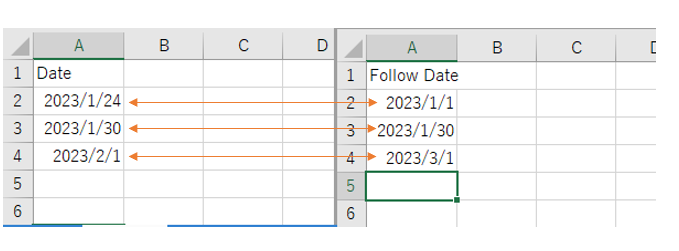# Compare two Different Excel with Different headers

HI Guys,
Im facing a error in comparing the headers. can u find out wat mistake i done. Any suggestion pls

Syntax i used to compare 2 excel file

Hi,

If you need to compare value of “Date” and “Follow Date” in same row index b/w two datatable, the following will work

``````dt1.AsEnumerable.Where(Function(r,i) Cdate(r("Date")).Equals(Cdate(dt2.Rows(i)("Follow Date")))).copytodatatable
``````

Regards,

1 Like

its not same row its different sheet and different excel

Hi,

I mean “same row index” is as the following image. Is this what you expect?If yes, the above expression will work.
if not, can you share your specific input and expected output? It’s no problem if dummy data.

Regards,

Both of the indexes are different.

@Rahul_PK

If you want to compare with each data in dt2 then use this expression inside for each datatable and loop dt2

now the formula would be

`dt1.Asenmerable.where(Function(x) Cdate(x(“Date”)).Equals(Cdate(Currentrow(“Follow Date”)))).copytodatatable`

This will give you rows matched in dt with the provided date in dt2

1 Like

Hi,

If you want to extract rows of dt1 which has same value in “Follow date” of dt2, the following will work.

``````dt1.AsEnumerable.Where(Function(r) dt2.AsEnumerable.Any(Function(r2)  Cdate(r("Date")).Equals(Cdate(r2("Follow Date"))))).copytodatatable
``````

If the above doesn’t work, can you share specific input and expected output?

Regards,

1 Like

you can use the Vlook up activity to matches the data

1 Like

Tqs Anil I got it

1 Like

This topic was automatically closed 3 days after the last reply. New replies are no longer allowed.